Slab + interval stats and geoms

Introduction

This vignette describes the slab+interval geoms and stats in ggdist. This is a flexible family of stats and geoms designed to make plotting distributions (such as priors and posteriors in Bayesian models, or even sampling distributions from other models) straightforward, and support a range of useful plots, including intervals, eye plots (densities + intervals), CCDF bar plots (complementary cumulative distribution functions + intervals), gradient plots, and histograms.

Setup

The following libraries are required to run this vignette:

library(dplyr)
library(tidyr)
library(distributional)
library(ggdist)
library(ggplot2)
library(cowplot)

theme_set(theme_ggdist())

Roadmap: It all starts with “slabinterval”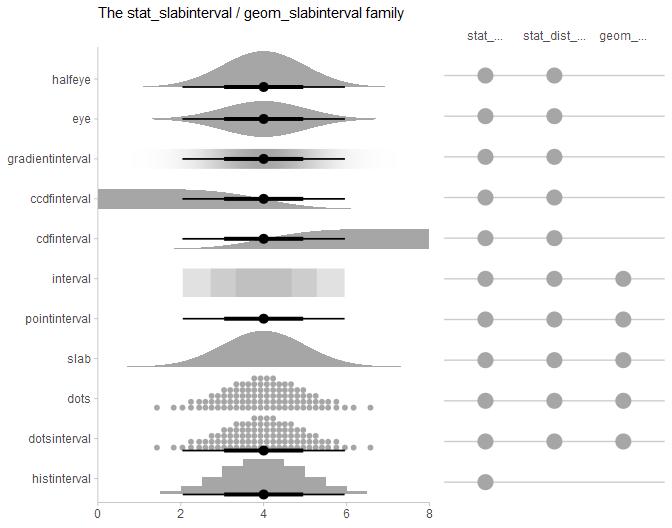ggdist has a pantheon of geoms and stats that stem from a common root: geom_slabinterval() and stat_slabinterval(). These geoms consist of a “slab” (say, a density or a CDF), one or more intervals, and a point summary. These components may be computed in a number of different ways, and different variants of the geom will or will not include all components.

Using geom_slabinterval() and stat_slabinterval() directly is not necessarily advisable: they are highly configurable on their own, but this configurability requires remembering a bunch of combinations of options to use. Instead, ggdist contains a number of pre-configured, easier-to-remember stats and geoms built on top of the slabinterval. These follow the following naming scheme:

[geom|stat|stat_dist]_[name]

For example, stat_dist_eye(), stat_eye(), stat_pointinterval(), geom_pointinterval(), etc. The naming scheme works as follows:

• Geoms starting with geom_ are meant to be used on already-summarized data (typically data summarized into intervals): things like geom_pointinterval() and geom_interval().
• Stats starting with stat_ are meant to be used on sample data; e.g. draws from a posterior distribution (or any other distribution, really). These stats compute relevant summaries (densities, CDFs, points, and/or intervals) before forwarding the summaries to their geom. Some have geom counterparts (e.g. stat_interval() corresponds to geom_interval(), except the former applies to sample data and the latter to already-summarized data). Many of these stats do not currently have geom counterparts (e.g. stat_ccdfinterval()), as they are primarily differentiated based on what kind of statistical summary they compute. If you’ve already computed a function (such as a density or CDF), you can just use geom_slabinterval() directly.
• Stats starting with stat_dist_ can be used to create slab+interval geoms for analytical distributions. They take either distributional objects or distribution names (the dist aesthetic) and arguments (the args aesthetic or arg1, … arg9 aesthetics) and compute the relevant slabs and intervals. Thus, where stat_eye() makes an eye plot for sample data, stat_dist_eye() makes an eye plot for an analytical distribution.

All slabinterval geoms can be plotted horizontally or vertically. Depending on how aesthetics are mapped, they will attempt to automatically determine the orientation; if this does not produce the correct result, the orientation can be overridden by setting orientation = "horizontal" or orientation = "vertical".

We’ll start with one of the most common existing use cases for these kinds geoms: eye plots.

Eye plots and half-eye plots

On sample data: stat_[half]eye

Eye plots combine densities (as violins) with intervals to give a more detailed picture of uncertainty than is available just by looking at intervals.

For these first few demos we’ll use these data:

set.seed(1234)
df = tribble(
~group, ~subgroup, ~value,
"a",          "h", rnorm(1000, mean = 5),
"b",          "h", rnorm(1000, mean = 7, sd = 1.5),
"c",          "h", rnorm(1000, mean = 8),
"c",          "i", rnorm(1000, mean = 9),
"c",          "j", rnorm(1000, mean = 7)
) %>%
unnest(value)

We can summarize it at the group level using an eye plot with stat_eye() (ignoring subgroups for now):

df %>%
ggplot(aes(y = group, x = value)) +
stat_eye() +
ggtitle("stat_eye()")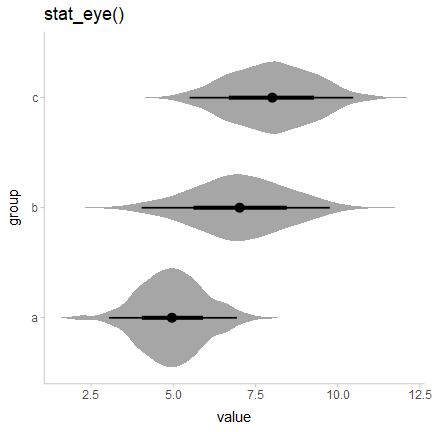Users of older versions of tidybayes (which used to contain the ggdist geoms) might have used geom_eye(), which is the older spelling of stat_eye(). Due to the name standardization in version 2 of tidybayes (see the description above), stat_eye() is now the preferred spelling. geom_eye() will continue to work for now, but is deprecated and may throw a warning in future versions.

We can also use stat_halfeye() instead to get densities instead of violins:

df %>%
ggplot(aes(y = group, x = value)) +
stat_halfeye() +
ggtitle("stat_halfeye()")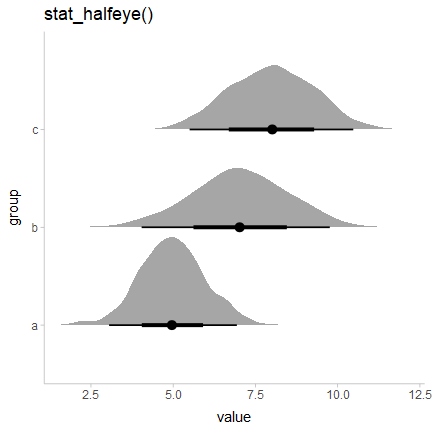Or use the side parameter to more finely control where the slab (in this case, the density) is drawn:

p = df %>%
ggplot(aes(x = group, y = value)) +
panel_border()

plot_grid(ncol = 3, align = "hv",
p + stat_eye(side = "left") + labs(title = "stat_eye()", subtitle = "side = 'left'"),
p + stat_eye(side = "both") + labs(subtitle = "side = 'both'"),
p + stat_eye(side = "right")  + labs(subtitle = "side = 'right'")
)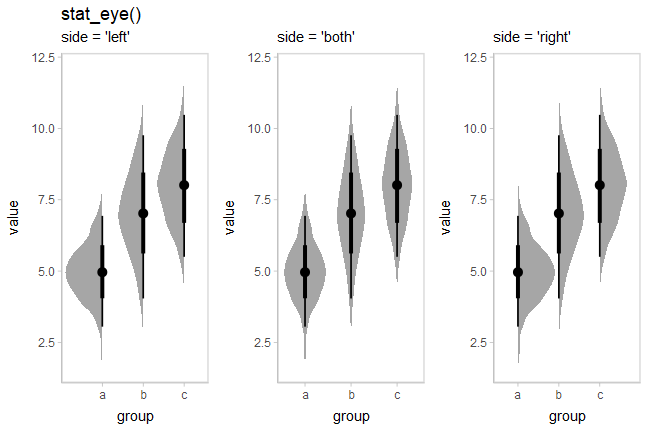Note how the above chart was drawn vertically instead of horizontally: all slabinterval geoms automatically detect their orientation based on the input data. For example, if you use a factor on one axis (say the x axis below), the geom will be drawn along the other axis:

df %>%
ggplot(aes(x = group, y = value)) +
stat_halfeye() +
ggtitle("stat_halfeye()")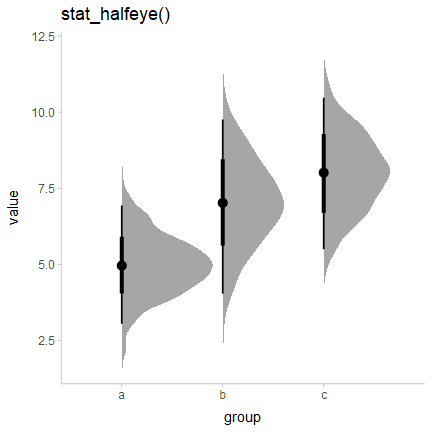If automatic detection of the desired axis fails, you can specify it manually; e.g. with stat_halfeye(orientation = 'vertical') or stat_halfeye(orientation = 'horizontal').

The side parameter works for horizontal geoms as well. "top" and "right" are considered synonyms, as are "bottom" and "left"; either form works with both horizontal and vertical versions of the geoms:

p = df %>%
ggplot(aes(x = value, y = group)) +
panel_border()

plot_grid(ncol = 3, align = "hv",
# side = "left" would give the same result
p + stat_eye(side = "left") + ggtitle("stat_eye()") + labs(subtitle = "side = 'bottom'"),
p + stat_eye(side = "both") + labs(subtitle = "side = 'both'"),
# side = "right" would give the same result
p + stat_eye(side = "right") + labs(subtitle = "side = 'top'")
)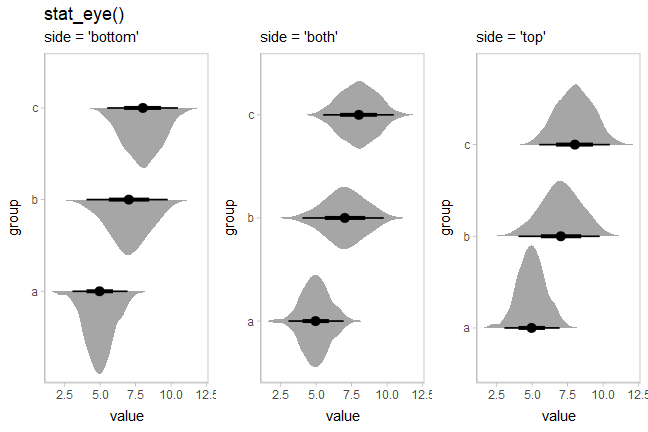Eye plots are also designed to support dodging through the standard mechanism of position = "dodge". Unlike with geom_violin(), densities in groups that are not dodged (here, ‘a’ and ‘b’) have the same area and max width as those in groups that are dodged (‘c’):

df %>%
ggplot(aes(x = group, y = value, fill = subgroup)) +
stat_eye(position = "dodge") +
ggtitle("stat_eye(position = 'dodge')")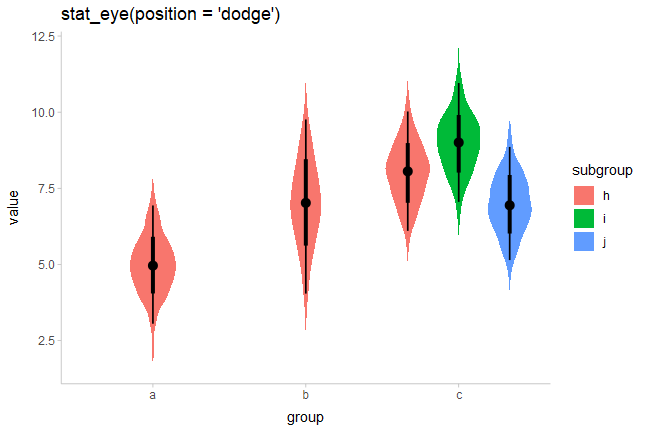Dodging works whether geoms are horizontal or vertical.

On analytical distributions: stat_dist_[half]eye

The same set of (half-)eye plot stats designed for sample data described above all have corresponding stats for analytical distributions: simply use stat_dist_ instead of stat_ in the name. These stats accept specifications for distributions using the dist and args aesthetics in one of two ways:

Using distribution names as character vectors: this format uses aesthetics as follows:

• dist: the name of the distribution, following R’s naming scheme. This is a string which should have "p", "q", and "d" functions defined for it: e.g., “norm” is a valid distribution name because the pnorm(), qnorm(), and dnorm() functions define the CDF, quantile function, and density function of the Normal distribution.
• args or arg1, … arg9: arguments for the distribution. If you use args, it should be a list column where each element is a list containing arguments for the distribution functions; alternatively, you can pass the arguments directly using arg1, … arg9.

Using distribution vectors from the distributional package: this format uses aesthetics as follows:

• dist: a distribution vector produced by functions such as distributional::dist_normal(), distributional::dist_beta(), etc.

For example, here are a variety of normal distributions describing the same data from the previous example:

dist_df = tribble(
~group, ~subgroup, ~mean, ~sd,
"a",          "h",     5,   1,
"b",          "h",     7,   1.5,
"c",          "h",     8,   1,
"c",          "i",     9,   1,
"c",          "j",     7,   1
)

We can visualize these distributions directly using stat_dist_eye() and the character vector input style to the dist, arg1, and arg2 aesthetics:

dist_df %>%
ggplot(aes(x = group, dist = "norm", arg1 = mean, arg2 = sd, fill = subgroup)) +
stat_dist_eye(position = "dodge") +
ggtitle("stat_dist_eye(position = 'dodge')")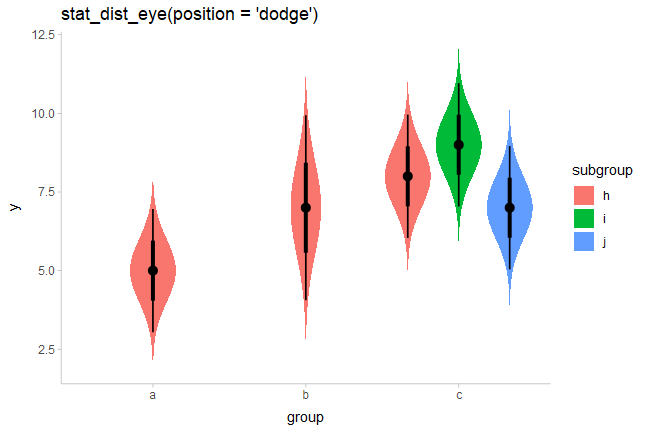Or we can use the distributional::dist_normal() function to construct a vector of normal distributions. This syntax is often more compact and expressive than the character-vector format above:

dist_df %>%
ggplot(aes(x = group, dist = dist_normal(mean, sd), fill = subgroup)) +
stat_dist_eye(position = "dodge") +
ggtitle("stat_dist_eye(position = 'dodge')")This makes it easy to visualize a variety of distributions. E.g., here are some Beta distributions:

data.frame(alpha = seq(5, 100, length.out = 10)) %>%
ggplot(aes(y = alpha, dist = dist_beta(alpha, 10))) +
stat_dist_halfeye() +
labs(
title = "stat_dist_halfeye()",
x = "Beta(alpha,10) distribution"
)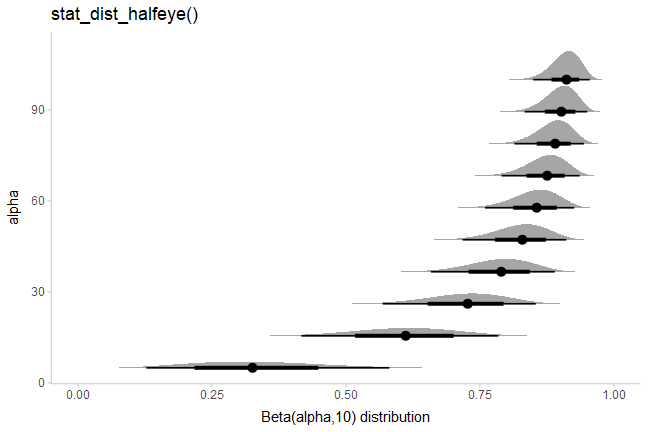If you want to plot all of these on top of each other (instead of stacked), you could turn off plotting of the interval to make the plot easier to read using stat_dist_halfeye(show_interval = FALSE, ...). A shortcut for stat_dist_halfeye(show_interval = FALSE, ...) is stat_dist_slab(). We’ll also turn off the fill color with fill = NA to make the stacking easier to see, and use outline color to show the value of alpha:

data.frame(alpha = seq(5, 100, length.out = 10)) %>%
ggplot(aes(y = "", dist = dist_beta(alpha, 10), color = alpha)) +
stat_dist_slab(fill = NA) +
coord_cartesian(expand = FALSE) +
scale_color_viridis_c() +
labs(
title = "stat_dist_slab()",
subtitle = "aes(dist = dist_beta(alpha, 10), color = alpha)",
x = "Beta(alpha,10) distribution",
y = NULL
)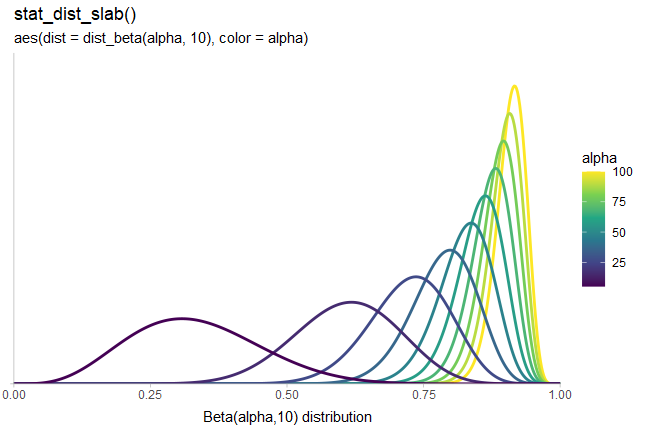The approach of using arg1, … arg9 can work well when comparing similar distributions, but is harder to use with different distribution types. For example, if we wished to compare a Student t distribution and Normal distribution, the arguments may not line up. This is a good case to use list columns and the args aesthetic. ggdist includes an implementation of the scaled and shifted Student t distribution (dstudent_t(), pstudent_t(), etc) as it is often needed for visualizing frequentist confidence distributions (see vignette("freq-uncertainty-vis")) and Bayesian priors:

tribble(
~ dist,      ~ args,
"norm",      list(0, 1),
"student_t", list(3, 0, 1)
) %>%
ggplot(aes(y = dist, dist = dist, args = args)) +
stat_dist_halfeye() +
ggtitle("stat_dist_halfeye()")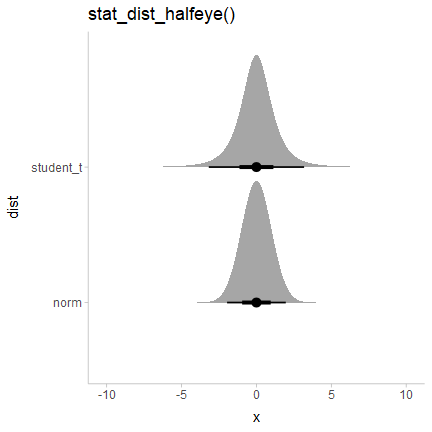A particularly good use of the dist stats is to visualize priors. For example, with brms you can specify priors using the brms::prior() function, which creates data frames with a "prior" column indicating the name of the prior distribution as a string. E.g., I might set some priors on the betas and the standard deviation in a model with something like this:

# NB these priors are made up!
priors = c(
prior(normal(0,1), class = b),
prior(lognormal(0,1), class = sigma)
)
priors
prior class coef group resp dpar nlpar bound
normal(0, 1) b
lognormal(0, 1) sigma

The parse_dist function can make it easier to visualize these: it takes in string specifications like those produced by brms"normal(0,1)" and "lognormal(0,1)" above — and translates them into .dist and .args columns:

priors %>%
parse_dist(prior)
prior class coef group resp dpar nlpar bound .dist .args
normal(0, 1) b norm 0, 1
lognormal(0, 1) sigma lnorm 0, 1

Notice that it also automatically translate some common distribution names (e.g. “normal” and “lognormal”) into their equivalent R function names ("norm" and "lnorm"). This makes it easy to use them with stat_dist_eye() and its variants:

priors %>%
parse_dist(prior) %>%
ggplot(aes(y = class, dist = .dist, args = .args)) +
stat_dist_halfeye() +
labs(
title = "stat_dist_halfeye()",
subtitle = "with brms::prior() and ggdist::parse_dist() to visualize priors",
x = NULL
)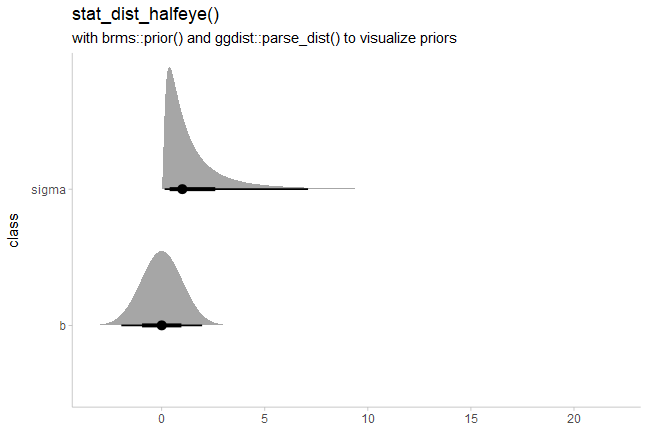The stat_dist_... family also adjusts densities appropriately when scale transformations are applied. For example, here is a log-Normal distribution plotted on a log scale:

data.frame(dist = "lnorm") %>%
ggplot(aes(y = 0, dist = dist, arg1 = log(10), arg2 = 2*log(10))) +
stat_dist_halfeye() +
scale_x_log10(breaks = 10^seq(-5,7, by = 2))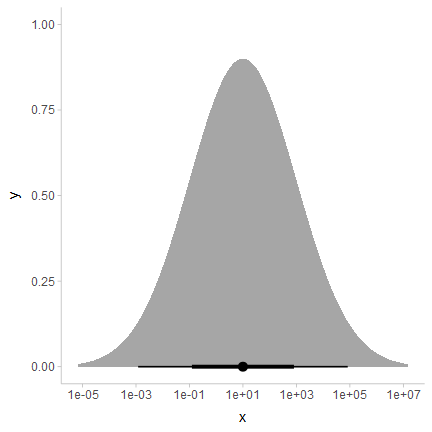As expected, a log-Normal density plotted on the log scale appears Normal. The Jacobian for the scale transformation is applied to the density so that the correct density is shown on the log scale. Internally, numerical differentiation is used to calculate the Jacobian so that the stat_dist_... family works generically across the different scale transformations supported by ggplot.

Summing up eye plots: stat_[dist_][half]eye

All of the above geoms follow the naming scheme stat_[dist_][half]eye.

• Add dist_ to the name to get stats for analytical distributions (otherwise it is for sample data).
• Add half to the name to get half-eyes (densities) instead of eyes (violins).

Histogram + interval plots

In some cases you might prefer histograms to density plots. stat_histinterval provides an alternative to stat_halfeye that uses histograms instead of densities:

p = df %>%
ggplot(aes(x = group, y = value)) +
panel_border()

ph = df %>%
ggplot(aes(y = group, x = value)) +
panel_border()

plot_grid(ncol = 2, align = "hv",
p + stat_histinterval() + labs(title = "stat_histinterval()", subtitle = "horizontal"),
ph + stat_histinterval() + labs(subtitle = "vertical")
)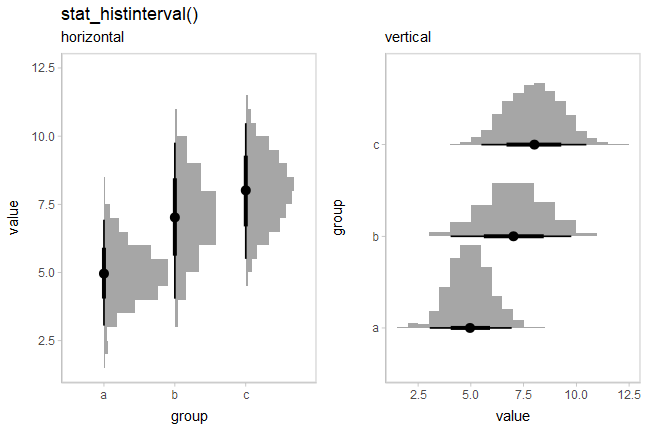You can use the slab_color aesthetic to show the outline of the bars. By default the outlines are only drawn on top of the bars, as typical tasks with histograms involve area estimation, so the outlines between bars are not strictly necessary and may be distracting. However, if you wish to include those outlines, you can set outline_bars = TRUE:

plot_grid(ncol = 2, align = "hv",
ph + stat_histinterval(slab_color = "gray45", outline_bars = FALSE) +
labs(title = "stat_histinterval", subtitle = "outline_bars = FALSE (default)"),
ph + stat_histinterval(slab_color = "gray45", outline_bars = TRUE) +
labs(subtitle = "outline_bars = TRUE")
)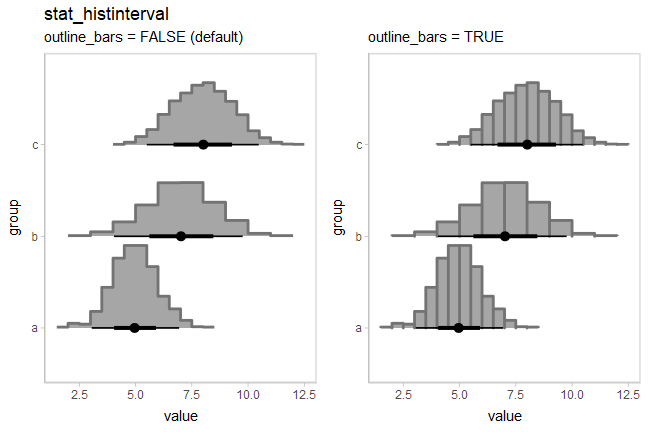There are currently no analytical (stat_dist_) versions of stat_histinterval().

CCDF bar plots

Another (perhaps sorely underused) technique for visualizing distributions is cumulative distribution functions (CDFs) and complementary CDFs (CCDFs). These can be more effective for some decision-making tasks than densities or intervals, and require fewer assumptions to create from sample data than density plots.

For all of the examples above, both on sample data and analytical distributions, you can replace [half]eye with [c]cdfinterval to get a stat that creates a CDF or CCDF bar plot.

On sample data: stat_[c]cdfinterval

stat_[c]cdfinterval has the following basic combinations:

p = df %>%
ggplot(aes(x = group, y = value)) +
panel_border()

ph = df %>%
ggplot(aes(y = group, x = value)) +
panel_border()

plot_grid(ncol = 2, align = "hv",
p + stat_ccdfinterval() + labs(title = "stat_ccdfinterval()", subtitle = "vertical"),
ph + stat_ccdfinterval() + labs(subtitle = "horizontal"),
p + stat_cdfinterval() + labs(title = "stat_cdfinterval()", subtitle = "vertical"),
ph + stat_cdfinterval()  + labs(subtitle = "horizontal")
)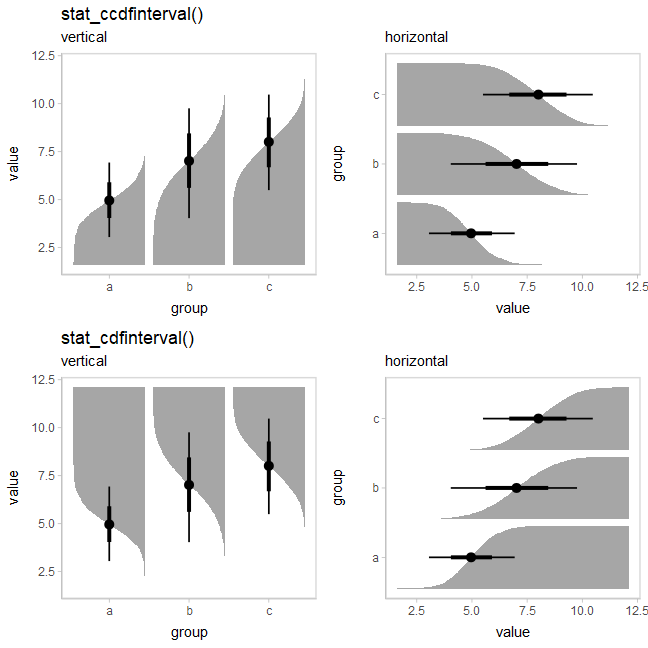The CCDF interval plots are probably more useful than the CDF interval plots in most cases, as the bars typically grow up from the baseline. For example, replacing stat_eye() with stat_ccdfinterval() in our previous subgroup plot produces CCDF bar plots:

df %>%
ggplot(aes(x = group, y = value, fill = subgroup, group = subgroup)) +
stat_ccdfinterval(position = "dodge") +
ggtitle("stat_ccdfinterval(position = 'dodge')")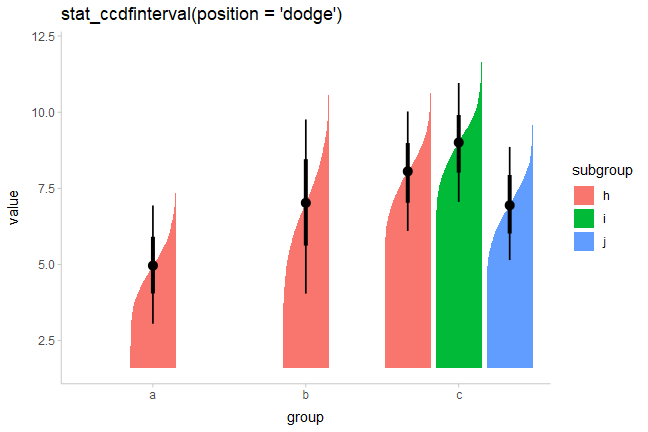The extents of the bars are determined automatically by range of the data in the samples. However, for bar charts it is often good practice to draw the bars from a meaningful reference point (this point is often 0). You can use ggplot2::expand_limits() to ensure the bar is drawn down to 0:

df %>%
ggplot(aes(x = group, y = value, fill = subgroup)) +
stat_ccdfinterval(position = "dodge") +
expand_limits(y = 0) +
# plus coord_cartesian so there is no space between bars and axis
coord_cartesian(expand = FALSE) +
ggtitle("stat_ccdfinterval(position = 'dodge')")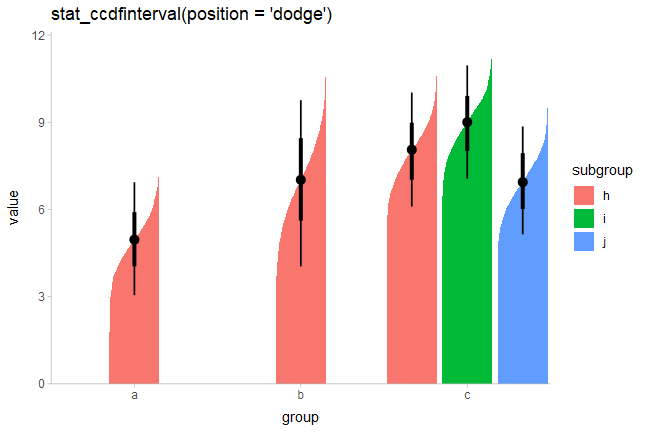You can also adjust the position of the slab relative to the position of the interval using the justification parameter:

df %>%
ggplot(aes(x = group, y = value, fill = subgroup)) +
stat_ccdfinterval(position = "dodge", justification = 1) +
expand_limits(y = 0) +
# clip = "off" needed here to ensure interval at the edge is visible
coord_cartesian(expand = FALSE, clip = "off") +
ggtitle("stat_ccdfinterval(position = 'dodge', justification = 1)")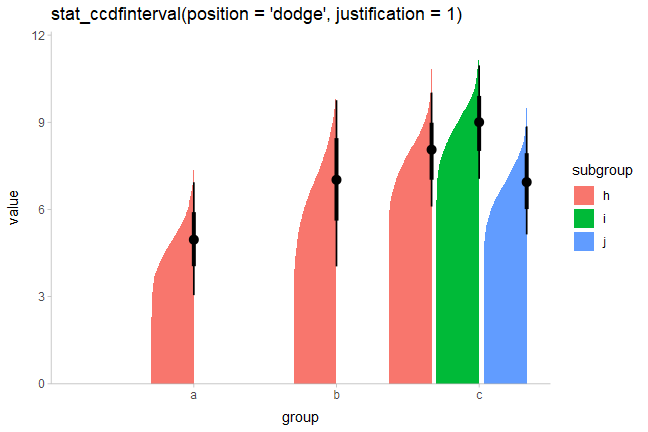The side parameter also works in the same way it does with stat_eye(). Here we’ll demonstrate it horizontally:

p = df %>%
ggplot(aes(x = value, y = group)) +
expand_limits(x = 0) +
panel_border()

plot_grid(ncol = 3, align = "hv",
# side = "left" would give the same result
p + stat_ccdfinterval(side = "bottom") + ggtitle("stat_ccdfinterval()") + labs(subtitle = "side = 'bottom'"),
p + stat_ccdfinterval(side = "both") + labs(subtitle = "side = 'both'"),
# side = "right" would give the same result
p + stat_ccdfinterval(side = "top") + labs(subtitle = "side = 'top'")
)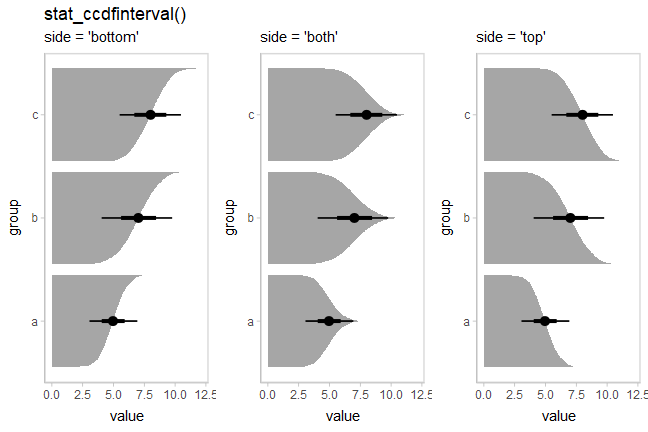On analytical distributions: stat_dist_[c]cdfinterval

You can also use stat_dist_ccdfinterval() instead if you wish to visualize analytical distributions, just as you can use stat_dist_eye().

By default, stat_dist_ccdfinterval() uses the quantiles at p = 0.001 and p = 0.999 of the distributions to determine their extent (unless the lower or upper limit of the distribution’s support is finite, in which case that value is used). You can change this setting using the p_limits parameter, or use expand_limits() to ensure a particular value is shown, as before:

dist_df %>%
ggplot(aes(x = group, dist = dist_normal(mean, sd), fill = subgroup)) +
stat_dist_ccdfinterval(position = "dodge") +
expand_limits(y = 0) +
ggtitle("stat_dist_ccdfinterval(position = 'dodge')") +
coord_cartesian(expand = FALSE)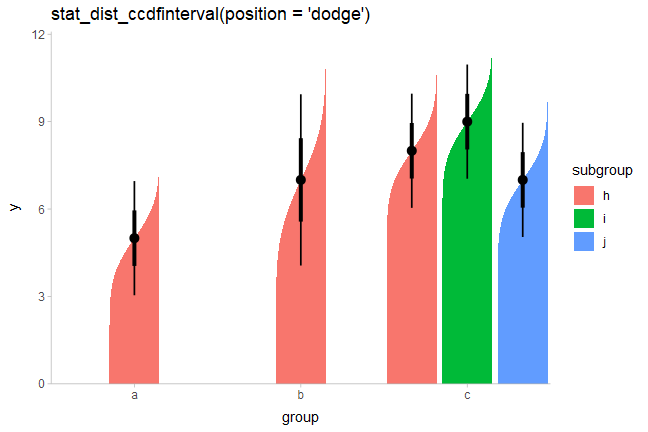Summing up CDF bar plots: stat_[dist_][c]cdfinterval

All of the above geoms follow the naming scheme stat_[dist_][c]cdfinterval.

• Add dist_ to the name to get stats for analytical distributions (otherwise it is for sample data).
• Add c to the name to get CCDFs instead of CDFs.

An alternative approach to mapping density onto the thickness aesthetic of the slab is to instead map it onto its alpha value (i.e., opacity). This is what the stat_[dist_]gradientinterval family does (actually, it uses slab_alpha, a variant of the alpha aesthetic, described below).

df %>%
ggplot(aes(x = group, y = value, fill = subgroup)) +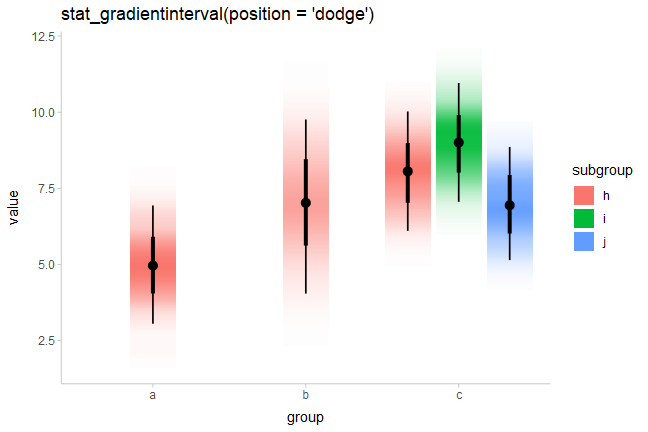Note on “choppy” gradients: Depending on your graphics device, gradients may be “choppy” looking. You can fix this choppiness by setting fill_type = "gradient", which uses an experimental gradient feature introduced in R 4.1 (in some future version of ggdist this is likely to become the default). This works so long as you have R version 4.1 or greater and you are using one of the graphics devices supported by the new grid::linearGradient() function (such as pdf(), svg(), or png(type = "cairo"); see here):

df %>%
ggplot(aes(x = group, y = value, fill = subgroup)) +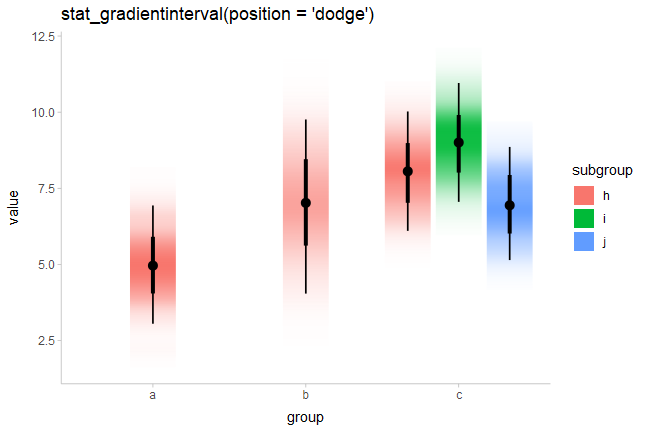stat_gradientinterval() maps density onto the slab_alpha aesthetic, which is a variant of the ggplot alpha scale that specifically targets alpha (opacity) values of the slab portion of geom_slabinterval(). This aesthetic has default ranges and limits that are a little different from the base ggplot alpha scale and which ensure that densities of 0 are mapped onto opacities of 0. You can use scale_slab_alpha_continuous() to adjust this scale’s settings.

On analytical distributions: stat_dist_[c]cdfinterval

As with other plot types, you can also use stat_dist_gradientinterval() instead if you wish to visualize analytical distributions:

dist_df %>%
ggplot(aes(x = group, dist = dist_normal(mean, sd), fill = subgroup)) +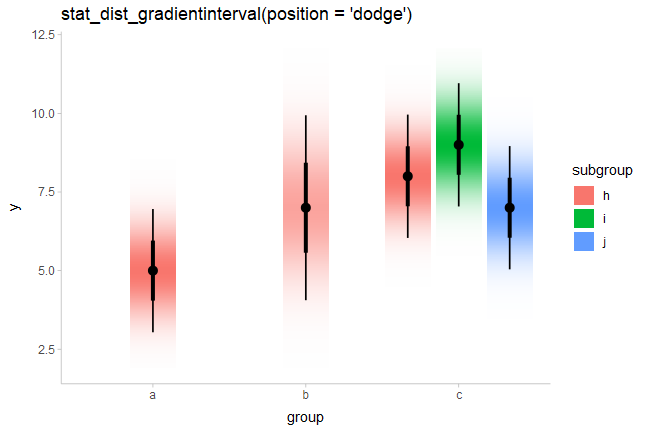• Add dist_ to the name to get stats for analytical distributions (otherwise it is for sample data).
• Add h to the name to get the horizontal version.

Dotplots

The encodings thus far are continuous probability encodings: they map probabilities or probability densities onto aesthetics like x/y position or transparency. An alternative is discrete or frequency-framing uncertainty visualizations, such as dotplots and quantile dotplots. These represent distributions as number of discrete possible outcomes.

On sample data: stat_dots

For example, replacing stat_halfeye() with stat_dots() produces dotplots. With so few dots here, the outlines mask the fill, so it makes sense to map the outline color of the dots as well:

df %>%
ggplot(aes(x = group, y = value, fill = subgroup, color = subgroup)) +
stat_dots(position = "dodge") +
labs(title = "stat_dots(aes(fill = subgroup, color = subgroup))")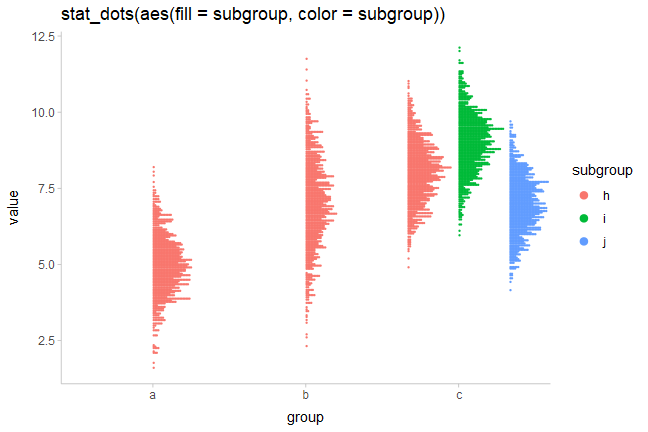Unlike the base ggplot2::geom_dotplot() geom, ggdist::geom_dots() automatically determines a bin width to ensure that the dot stacks fit within the available space.

Quantile dotplots

The above plots are a bit hard to read due to the large number of dots. Particularly when summarizing posterior distributions or predictive distributions, it can make sense to plot a smaller number of dots (say 20, 50 or 100) that are representative of the full sample. One such approach is to plot quantiles, thereby creating quantile dotplots, which can help people make decisions under uncertainty (Kay 2016, Fernandes 2018).

The quantiles argument to stat_dots constructs a quantile dotplot with the specified number of quantiles. Here is one with 50 quantiles, so each dot represents approximately a 2% (1/50) chance. We’ll turn off outline color too (color = NA):

df %>%
ggplot(aes(x = group, y = value, fill = subgroup)) +
stat_dots(position = "dodge", quantiles = 50, color = NA) +
labs(title = "stat_dots(quantiles = 50)")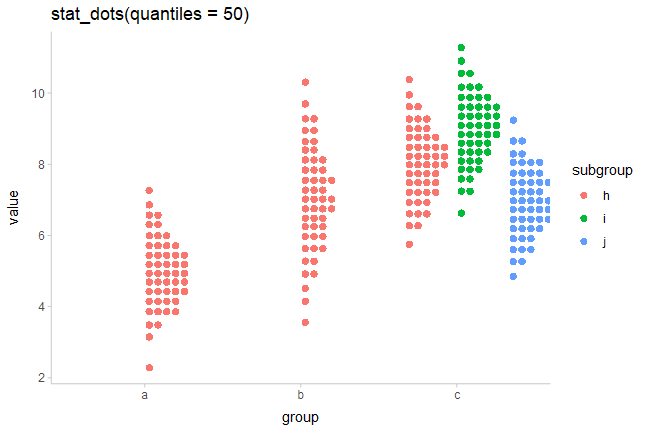On analytical distributions: stat_dist_dots

As with other plot types, you can also use stat_dist_dots() instead if you wish to visualize analytical distributions. Analytical dotplots default to 100-dot quantile dotplots (as above, this can be adjusted with the quantiles argument). Shapes of the dots can also be changed using the shape aesthetic, and as with all slabinterval geoms, fill and color aesthetics can be varied within the geoms, as demonstrated below (we’ll also put the group on the y axis to plot it horizontally):

dist_df %>%
filter(group != "c") %>%
ggplot(aes(y = group, dist = dist_normal(mean, sd), fill = stat(x < 5), shape = stat(x < 5))) +
stat_dist_dots(position = "dodge", color = NA) +
labs(title = "stat_dist_dots(aes(fill and shape = stat(x < 5)))") +
geom_vline(xintercept = 5, alpha = 0.25) +
scale_x_continuous(breaks = 2:10) +
# we'll use these shapes since they have fill and outlines
scale_shape_manual(values = c(21,22))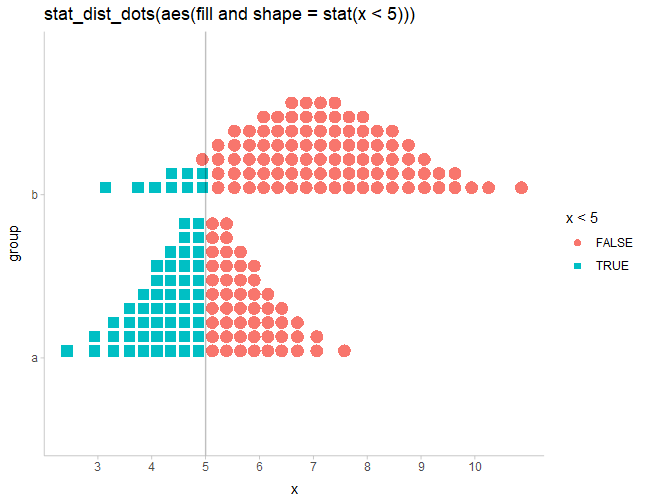Notice the default dotplot layout, "bin", can cause dots to be on the wrong side of a cutoff when coloring dots within dotplots. Thus it can be useful to use the "weave" layout, which positions dots at (or very close to) their true positions, rather than at bin centers:

dist_df %>%
filter(group != "c") %>%
ggplot(aes(y = group, dist = dist_normal(mean, sd), fill = stat(x < 5))) +
stat_dist_dots(position = "dodge", color = NA, layout = "weave") +
labs(title = 'stat_dist_dots(aes(fill = stat(x < 5)), layout = "weave")') +
geom_vline(xintercept = 5, alpha = 0.25) +
scale_x_continuous(breaks = 2:10)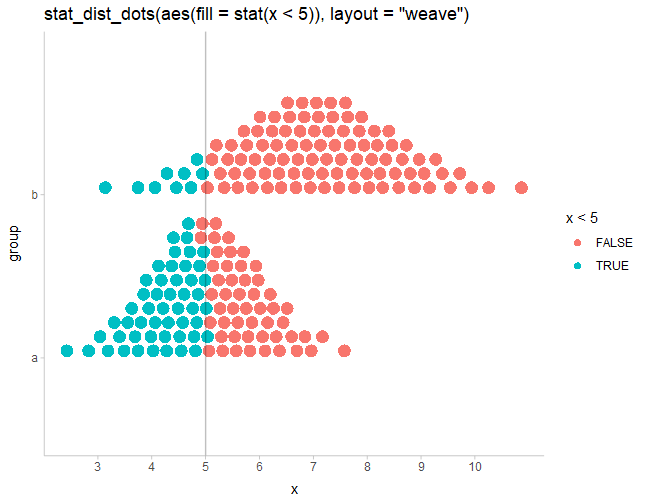As with other slabinterval geoms, the side argument can also be used to construct violin-style dotplots. This example also shows the use of dotsinterval in place of dots to construct a combined quantile dotplot violin + interval plot. We also set slab_color = NA to turn off the outline on the dots:

dist_df %>%
filter(group != "c") %>%
ggplot(aes(x = group, dist = dist_normal(mean, sd), fill = group)) +
stat_dist_dotsinterval(position = "dodge", side = "both", slab_color = NA) +
labs(title = "stat_dist_dotsinterval(side = 'both', slab_color = NA)")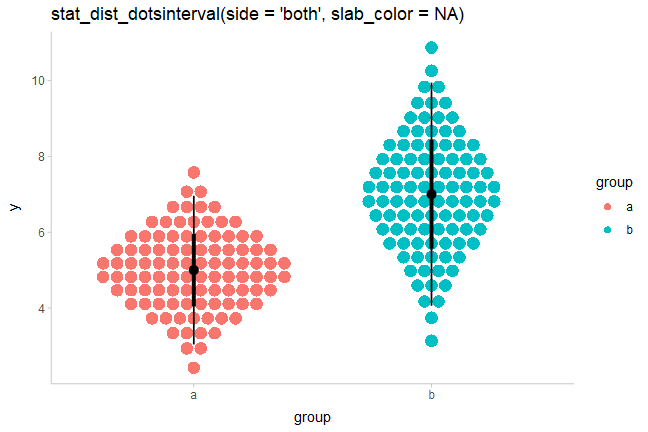Using beeswarm layouts

Finally, while the "bin" and "weave" layout methods shown above work especially well for quantile dotplots, it can be useful when visualizing sample data (especially in large amounts) to employ so-called “beeswarm” plots. Setting layout = "swarm" will use the "compactswarm" layout type from beeswarm::beeswarm(), which tends to work well on sample data (but not on quantiles), and especially when side = "both":

df %>%
filter(group == "a") %>%
ggplot(aes(y = group, x = value, fill = stat(x < 5))) +
stat_dots(color = NA, layout = "swarm", side = "both") +
labs(title = 'stat_dots(aes(fill = stat(x < 5)), layout = "swarm", side = "both")') +
geom_vline(xintercept = 5, alpha = 0.25) +
scale_x_continuous(breaks = 2:8)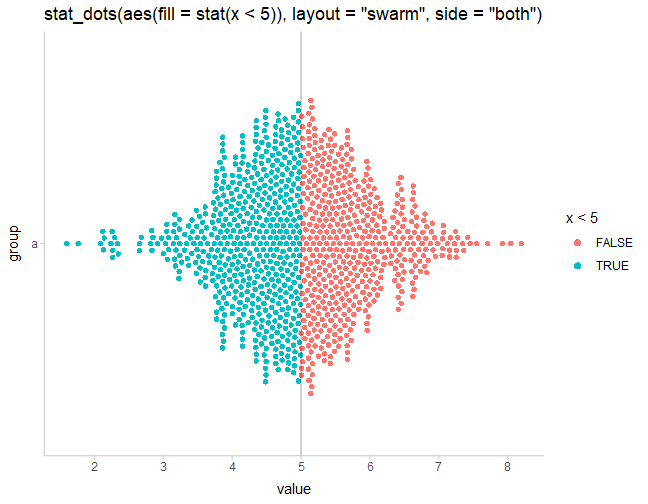Thus there are three layout options with dots and dotsinterval stats and geoms:

• layout = "bin", which maintains neat rows and columns and works well on quantile dotplots.
• layout = "weave", which maintains neat rows (but not columns) and works well on quantile dotplots when you need to map other aesthetics based on positional cutoffs.
• layout = "swarm", which does not maintain rows or columns, and works well when you need a compact layout for sample data (not quantiles).

Summing up dotplots: stat_[dist_]dots[interval]

All of the above geoms follow the naming scheme stat_[dist_]dots[interval].

• Add dist_ to the name to get stats for analytical distributions (otherwise it is for sample data).
• Add interval to the name to get the version with a point+interval geom overlaid.

Custom plots

The slabinterval family of stats and geoms is designed to be very flexible. Most of the shortcut geoms above can be created simply by setting particular combinations of options and aesthetic mappings using the basic geom_slabinterval(), stat_sample_slabinterval(), and stat_dist_slabinterval(). Some useful combinations do not have specific shortcut geoms currently, but can be created manually with only a bit of additional effort.

Gradients of alpha, color, and fill

Two aesthetics of particular use for creating custom geoms are slab_alpha, which changes the alpha transparency of the slab portion of the geom, slab_color, which changes its outline color, and fill, which changes its fill color. All of these aesthetics can be mapped to variables along the length of the geom (that is, the color does not have to be constant over the entire geom), which allows you to create gradients or to highlight meaningful regions of the data (amongst other things). You can also employ the ggdist-specific color_ramp and fill_ramp aesthetics to create custom gradients with outline and fill colors, as demonstrated later in this section.

Note: The examples of gradients in this section use the (optional) experimental setting fill_type = "gradient". If you do not have R greater than 4.1.0 or are not using a supported graphics device, the output may be blank; in this case, omit this option. Gradients can be produced without this option but they may not look as nice.

For example, stat_ccdfinterval() maps the output of the evaluated function (in its case, the CCDF) onto the thickness aesthetic of the slabinterval geom, which determines how thick the slab is. This is the equivalent of setting aes(thickness = stat(f)). However, we could instead create a CCDF gradient plot, a sort of mashup of a CCDF barplot and a density gradient plot, by mapping stat(f) onto the slab_alpha aesthetic instead, and setting thickness to a constant (1):

df %>%
ggplot(aes(x = group, y = value, fill = subgroup)) +
stat_ccdfinterval(aes(slab_alpha = stat(f)),
thickness = 1, position = "dodge", fill_type = "gradient"
) +
expand_limits(y = 0) +
# plus coord_cartesian so there is no space between bars and axis
coord_cartesian(expand = FALSE) +
ggtitle("stat_ccdfinterval(aes(slab_alpha = stat(f)), thickness = 1)")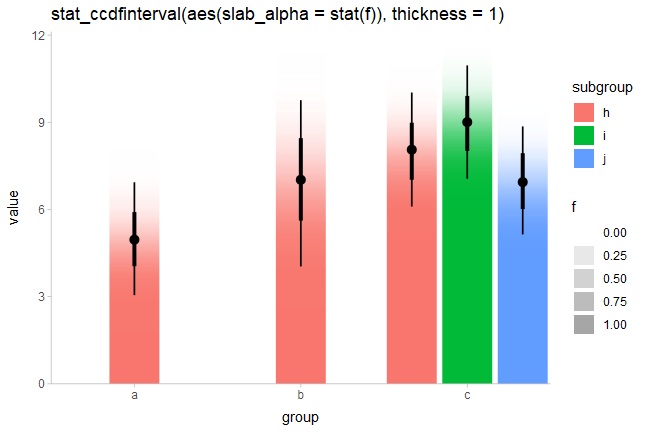If this approach were applied to bins in a histogram, where each bin had some uncertainty associated with its height, the result would be a so-called fuzzygram (Wilkinson 1992).

Highlighting and other combinations

The ability to map arbitrary variables onto fill or outline colors within a slab allows you to easily highlight sub-regions of a plot. Taking the earlier example of visualizing priors, we can add a mapping to the fill aesthetic to highlight a region of interest, say ±1.5:

priors = tribble(
~ dist,      ~ args,
"norm",      list(0, 1),
"student_t", list(3, 0, 1)
)

priors %>%
ggplot(aes(y = dist, dist = dist, args = args)) +
stat_dist_halfeye(aes(fill = stat(abs(x) < 1.5))) +
ggtitle("stat_dist_halfeye(aes(fill = stat(abs(x) < 1.5)))") +
# we'll use a nicer palette than the default for highlighting:
scale_fill_manual(values = c("gray85", "skyblue"))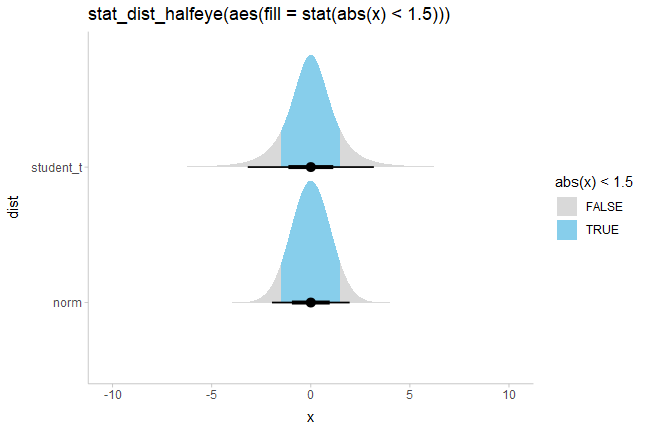We could also combine these aesthetics arbitrarily. Here is a (probably not very useful) eye plot + gradient plot combination, with the portion of the distribution above 1 highlighted:

priors %>%
ggplot(aes(y = dist, dist = dist, args = args)) +
stat_dist_eye(aes(slab_alpha = stat(f), fill = stat(x > 1)), fill_type = "gradient") +
ggtitle("stat_dist_eye(aes(slab_alpha = stat(f), fill = stat(x > 1)))") +
# we'll use a nicer palette than the default for highlighting:
scale_fill_manual(values = c("gray75", "skyblue"))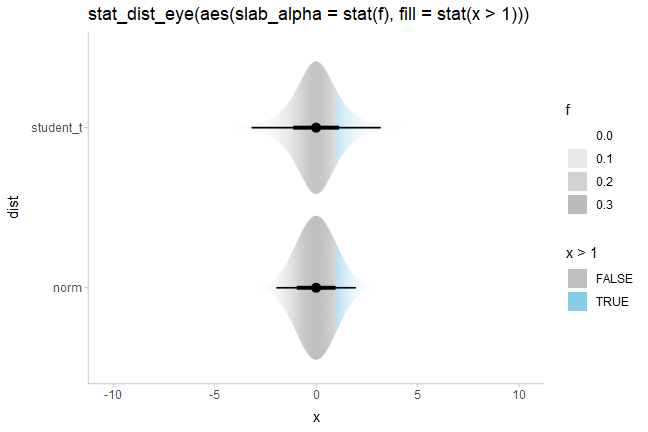Mashups with Correll and Gleicher-style gradients

We can also take advantage of the fact that all slabinterval stats also supply cdf and pdf aesthetics to create charts make use of both the CDF and the PDF in their aesthetic mappings. For example, we could create Correll & Gleicher-style gradient plots by fading the tails outside of the 95% interval in proportion to $$|1 - 2F(x)|$$ (where $$F(x)$$ is the CDF):

priors %>%
ggplot(aes(y = dist, dist = dist, args = args)) +
stat_dist_gradientinterval(aes(slab_alpha = stat(-pmax(abs(1 - 2*cdf), .95))),
) +
scale_slab_alpha_continuous(guide = "none")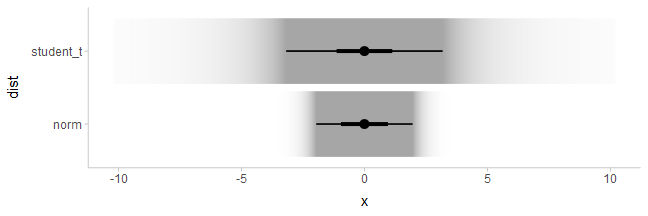We could also do a mashup of faded-tail gradients with violin plots by starting with an eye plot and then using the generated cdf aesthetic to fade the tails, producing plots like those in Helske et al.:

priors %>%
ggplot(aes(y = dist, dist = dist, args = args)) +
stat_dist_eye(aes(slab_alpha = stat(-pmax(abs(1 - 2*cdf), .95))), fill_type = "gradient") +
scale_slab_alpha_continuous(guide = "none")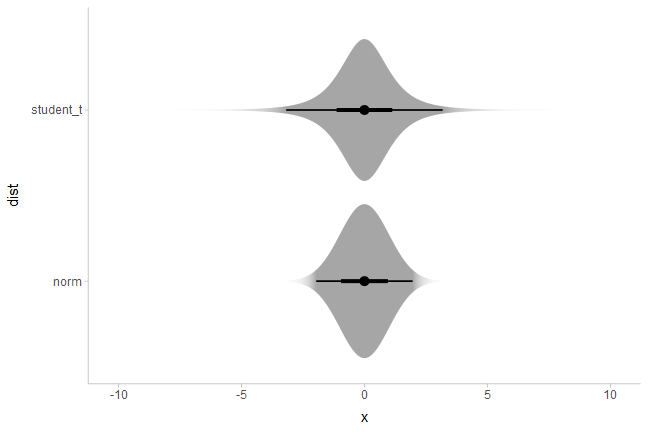A related idea is one from Tukey: rather than visually emphasizing where a value is likely, emphasize where it is unlikely. While Tukey used a visual representation showing both pointwise and simultaneous intervals, for this example we will do something a bit different, inverting the faded-tails function from Correll & Gleicher to create bars that “block out” the regions of low likelihood:

dist_df %>%
ggplot(aes(x = group, dist = dist_normal(mean, sd), fill = subgroup)) +
stat_dist_slab(aes(
thickness = stat(pmax(0, abs(1 - 2*cdf) - .95)),
fill_ramp = stat(pmax(0, abs(1 - 2*cdf) - .95))
),
side = "both", position = "dodge", fill_type = "gradient"
) +
labs(
title = 'stat_dist_slab(side = "both")',
subtitle = paste0(
"aes(fill = subgroup,\n       ",
"fill_ramp and thickness = stat(pmax(0, abs(1 - 2*cdf) - .95)))"
)
) +
guides(fill_ramp = "none") +
coord_cartesian(expand = FALSE)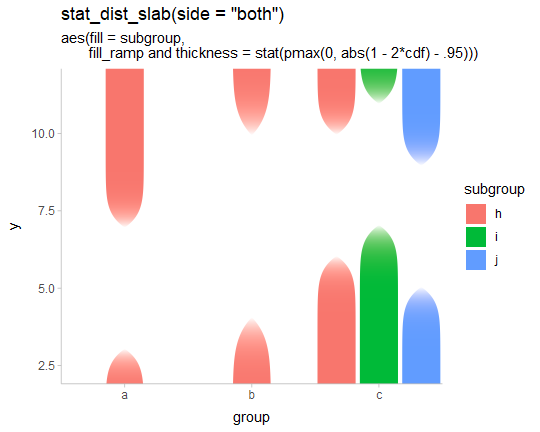Thanks to a tweet from Jessica Hullman that inspired the idea.

Densities filled according to intervals

Another common chart type involves filling in the interior of a halfeye plot according to some intervals. We can again use the fact that slabinterval stats auto-calculate both the PDF and CDF of a distribution as statistics. We can then the cut_cdf_qi() function, which labels points in a CDF according to which quantile interval they fall into, to determine the fill color:

df %>%
ggplot(aes(y = group, x = value)) +
stat_halfeye(aes(fill = stat(cut_cdf_qi(cdf)))) +
scale_fill_brewer(direction = -1) +
labs(
title = "stat_halfeye()",
subtitle = "aes(fill = stat(cut_cdf_qi(cdf)))",
fill = "Interval"
)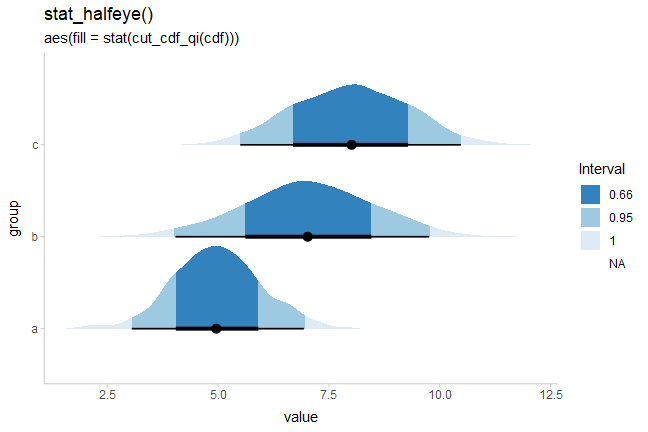Like point_interval(), cut_cdf_qi() takes a .width parameter to define the intervals, with a default of c(.66, .95, 1). The final 1 is necessary to show the full density, to cut off the tails of the density you can omit it. Like ggplot scales, cut_cdf_qi() also takes a labels parameter to define the labels of the intervals (or to provide a function to define the labels, such as a formatting function like scales::percent_format()). Let’s modify the intervals to show the 50%, 80%, and 95% intervals, omitting the tails of the density and reformatting the interval labels to use percentages. We can also use na.translate = FALSE to drop the unnecessary NA level from the fill scale:

df %>%
ggplot(aes(y = group, x = value)) +
stat_halfeye(aes(fill = stat(cut_cdf_qi(
cdf,
.width = c(.5, .8, .95),
labels = scales::percent_format()
)))) +
scale_fill_brewer(direction = -1, na.translate = FALSE) +
labs(
title = "stat_halfeye()",
subtitle = "aes(fill = stat(cut_cdf_qi(cdf, .width = c(.5, .8, .95))))",
fill = "Interval"
)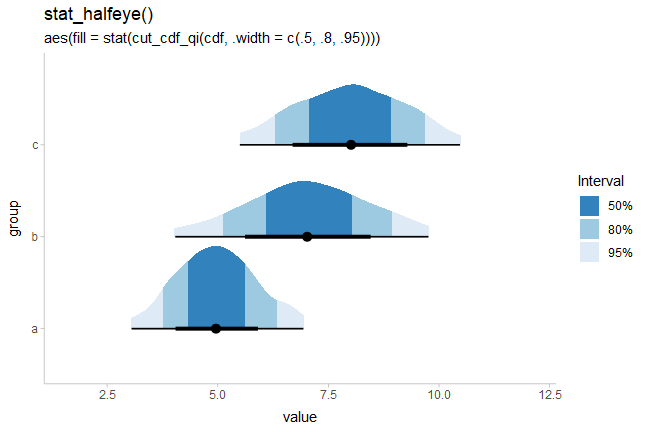Using color ramps for fill and color aesthetics

ggdist supplies color_ramp (or colour_ramp) and fill_ramp aesthetics which can be used to vary (“ramp”) the outline or fill colors smoothly from a base color (default "white") to whatever color the geometry would otherwise have.

Taking the above example with cut_cdf_qi(), we could use the fill_ramp aesthetic instead of the fill aesthetic to set the slab color based on the interval it is in. We could then vary the base fill color separately from the interval based on another column in the original data table, such as the subgroup column:

df %>%
ggplot(aes(y = group, x = value)) +
stat_halfeye(
aes(
fill = subgroup,
fill_ramp = stat(cut_cdf_qi(
cdf,
.width = c(.5, .8, .95),
labels = scales::percent_format()
))
),
# NOTE: we use position = "dodgejust" (a dodge that respects the
# justification of intervals relative to slabs) instead of
# position = "dodge" here because it ensures the topmost slab does
# not extend beyond the plot limits
position = "dodgejust",
) +
# a range from 1 down to 0.2 ensures the fill goes dark to light inside-out
# and doesn't get all the way down to white (0) on the lightest color
scale_fill_ramp_discrete(range = c(1, 0.2), na.translate = FALSE) +
labs(
title = "stat_halfeye()",
subtitle = "aes(fill = subgroup, fill_ramp = stat(cut_cdf_qi(cdf)))",
fill_ramp = "Interval"
)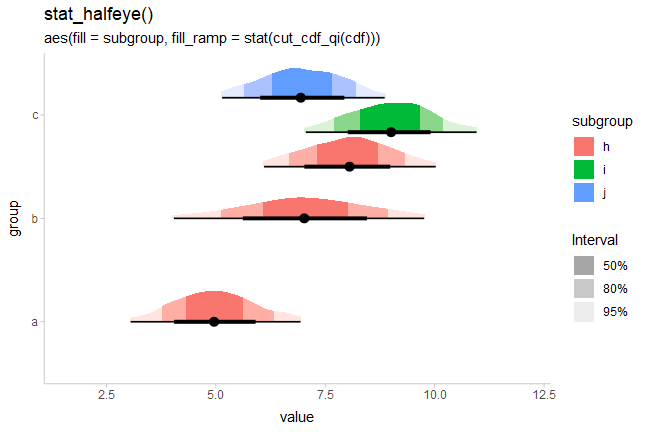We could similarly use the stat_dist_interval() (or stat_interval()) geometries with the color_ramp aesthetic to vary subgroup color separately from the whiteness of the intervals. Here, level is a variable generated by all stats in the stat_dist_... family which contains the level of the generated intervals, as an ordered factor.

dist_df %>%
ggplot(aes(x = group, dist = dist_normal(mean, sd), color = subgroup)) +
stat_dist_interval(aes(color_ramp = stat(level)), position = "dodge") +
labs(
title = "stat_dist_interval()",
subtitle = "aes(color = subgroup, color_ramp = stat(level))"
)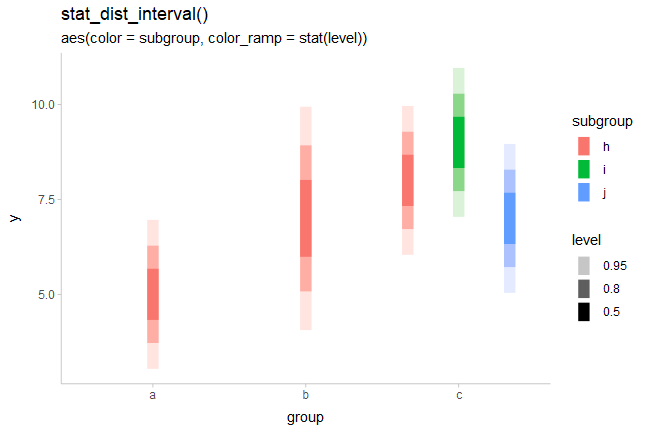Varying side, scale, and justification within geoms

The side, scale, and justification parameters can also be varied within a geom, allowing (for example) different groups to hang above or below the interval:

dist_df %>%
filter(subgroup == "h") %>%
mutate(side = c("top", "both", "bottom")) %>%
ggplot(aes(y = group, dist = dist_normal(mean, sd), side = side)) +
stat_dist_dotsinterval(scale = 2/3) +
labs(title = 'stat_dist_dotsinterval(aes(side = c("top","both","bottom")))') +
coord_cartesian()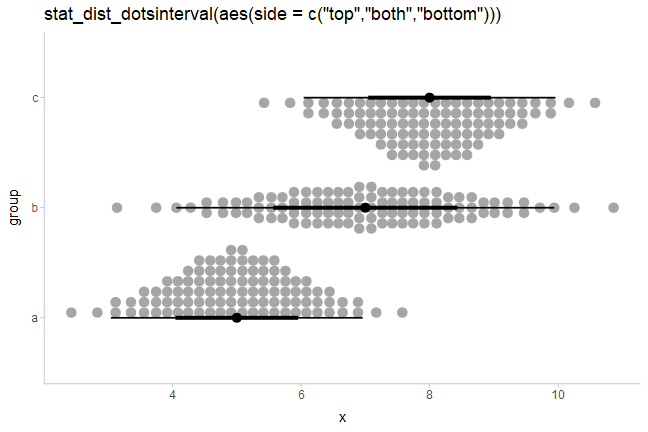Discrete distributions with redundant encodings

The stat_dist_... family will automatically detect discrete distributions and plot them using stepped histograms instead of densities. As with stat_histinterval(), you can choose whether or not to draw outlines between bars of the histogram using outline_bars = TRUE or FALSE (the default is FALSE). Here is an example of a redundant encoding of thickness and fill color on stepped histograms inspired by an example from Isabella Ghement:

tibble(
group = c("a","b","c","d","e"),
lambda = c(13,7,4,3,2)
) %>%
ggplot(aes(x = group)) +
stat_dist_slab(aes(dist = dist_poisson(lambda), fill = stat(pdf))) +
geom_line(aes(y = lambda, group = NA), size = 1) +
geom_point(aes(y = lambda), size = 2.5) +
labs(fill = "Pr(y)") +
ggtitle("stat_dist_slab()", "aes(dist = dist_poisson(lambda), fill = stat(pdf))")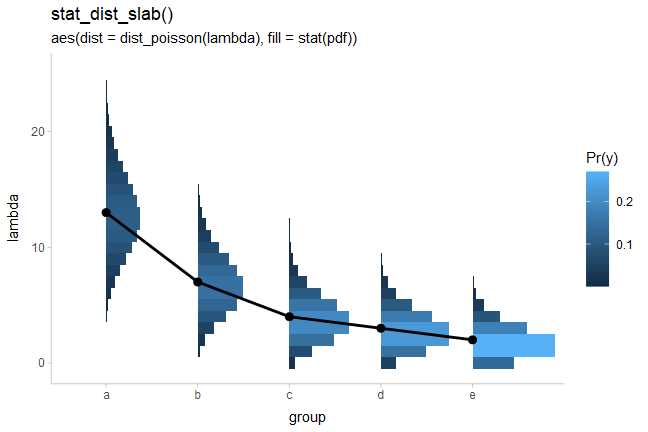Multiple slabs and intervals in composite plots

Sometimes you may want to include multiple different types of slabs in the same plot in order to take advantage of the features each slab type provides. For example, people often combine densities with dotplots to show the underlying datapoints that go into a density estimate, creating so-called “rain cloud” plots. To use multiple slab geometries together, you can use the side parameter to change which side of the interval a slab is drawn on and set the scale parameter to something around 0.5 (by default it is 0.9) so that the two slabs do not overlap. We’ll also scale the halfeye slab thickness by n (the number of observations in each group) so that the area of each slab represents sample size (and looks similar to the total area of its corresponding dotplot).

We’ll use a subsample of of the data to show how it might look on a reasonably-sized dataset.

set.seed(12345) # for reproducibility
df %>%
filter(subgroup == "h") %>%
group_by(group, subgroup) %>%
sample_n(100) %>%
ggplot(aes(y = group, x = value)) +
stat_slab(scale = 0.6, position = "dodge") +
stat_dotsinterval(side = "bottom", scale = 0.6, position = "dodge") +
labs(title = 'stat_slab(scale = 0.6) + \nstat_dotsinterval(scale = 0.6, side = "bottom")')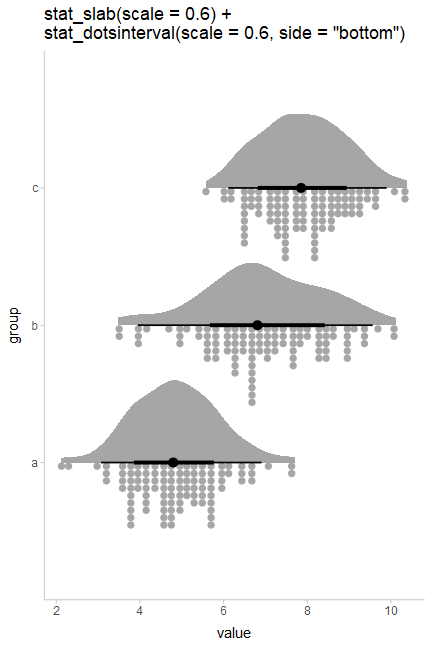Geoms can also be dodged together, as in this example using densities with quantile dotplots in subgroups. This example also shows how stat_pointinterval() can be repurposed to be used with other geoms; here to replace points with labels (the idea of replacing points with labels comes from Brenton Wiernik).

df %>%
ggplot(aes(x = group, y = value, fill = subgroup)) +
stat_slab(side = "left", scale = 0.5, position = "dodge") +
stat_dotsinterval(scale = 0.5, quantiles = 100, position = "dodge") +
stat_pointinterval(
geom = "label",
aes(label = paste0(group, subgroup)),
.width = .5,  # set to a scalar to draw only one label instead of two
position = position_dodge(width = 1),
size = 3.5
) +
labs(title = 'stat_halfeye(side = "left") + stat_dotsinterval(quantiles = 100) +\nstat_pointinterval(geom = "label")')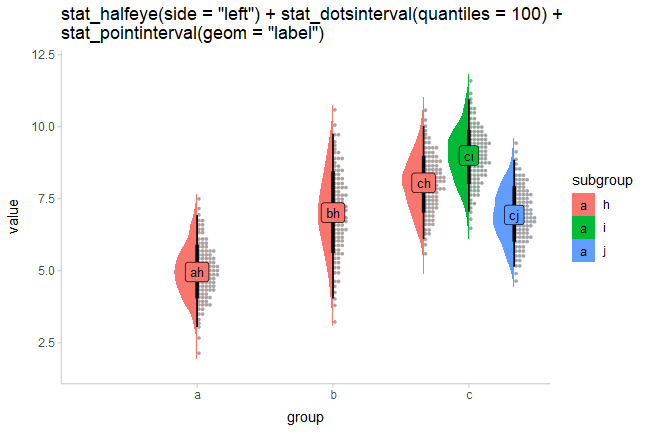When constructing composite plots it may be useful to position slab and interval parts of the geometry separately. While some relative positioning of these geometries is supported by manipulating the justification parameter, if you want complete, separate control over positioning of intervals versus slabs, the simplest approach can be to specify those geometries separately.

For example, the following uses a separate specification of a stat_slab() and a stat_pointinterval() instead of a combined stat_slabinterval() in order to use position_dodge() on the intervals but not the slabs:

df %>%
ggplot(aes(fill = group, color = group, x = value)) +
stat_slab(alpha = .3) +
stat_pointinterval(position = position_dodge(width = .4, preserve = "single")) +
labs(
title = "stat_slab() and stat_pointinterval()",
subtitle = "with position_dodge() applied to the intervals",
y = NULL
) +
scale_y_continuous(breaks = NULL)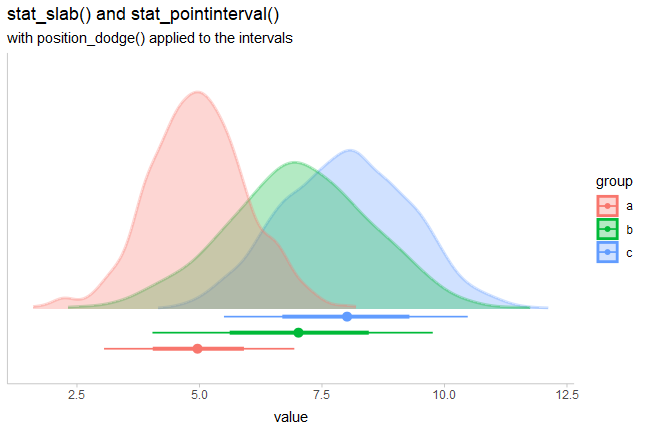(Thanks to Brenton Wiernik for this example.)